• +91 9971497814
• info@interviewmaterial.com

# RD Chapter 6- Graphs of Trigonometric Functions Ex-6.3 Interview Questions Answers

### Related Subjects

Question 1 :
Sketch the graphs of the following functions:
f (x) = 2 cosec πx

We know that f (x) = cosec x is a periodic function with period 2π.
So, f (x) = 2 cosec (πx) is a periodic function with period 2. So, we will draw the graph of f (x) = 2 cosec (πx) in the interval [0, 2]. The values of f (x) = 2 cosec (πx) at various points in [0, 2] are listed in the following table:
 x 0 (A) 1/2 (B) 1 (C) -1 (D) 3/2 (E) -2 (F) 2 (G) 5/2 (H) f (x) = 2 cosec (πx) ∞ 2 ∞ -∞ -2 -∞ ∞ 2

The required curve is: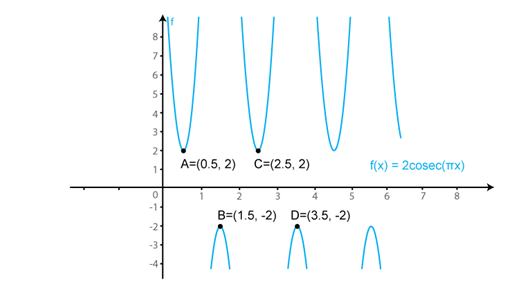Question 2 : f (x) = 3 sec x

We know that f (x) = sec x is a periodic function with period π.
So, f (x) = 3 sec (x) is a periodic function with period π. So, we will draw the graph of f (x) = 3 sec (x) in the interval [0, π]. The values of f (x) = 3 sec (x) at various points in [0, π] are listed in the following table:
 x 0 (A) π/2 (B) -π/2 (C) π (D) -3π/2 (E) 3π/2 (F) 2π (G) 5π/2 (H) f (x) = sec x 3 ∞ -∞ -3 -∞ ∞ 3 ∞

The required curve is: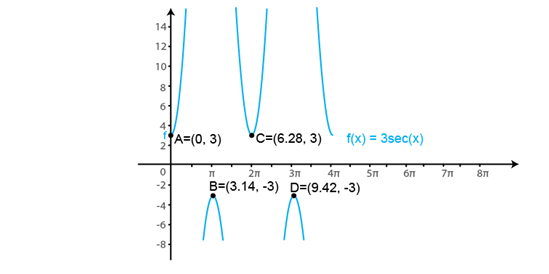Question 3 : f (x) = cot 2x

We know that f (x) = cot x is a periodic function with period π.
So, f (x) = cot (2x) is a periodic function with period π. So, we will draw the graph of f (x) = cot (2x) in the interval [0, π]. The values of f (x) = cot (2x) at various points in [0, π] are listed in the following table:
 x 0 (A) π/4 (B) -π/2 (C) π/2 (D) 3π/4 (E) -π (F) f (x) = cot x →∞ 0 -∞ →∞ 0 -∞

The required curve is: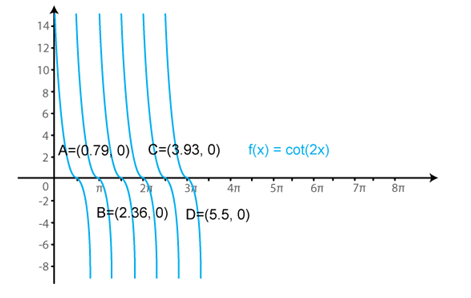Question 4 : f (x) = 2 sec πx

We know that f (x) = sec x is a periodic function with period π.
So, f (x) = 2 sec (πx) is a periodic function with period 1. So, we will draw the graph of f (x) = 2 sec (πx) in the interval [0, 1]. The values of f (x) = 2 sec (πx) at various points in [0, 1] are listed in the following table:
 x 0 1/2 -1/2 1 -3/2 3/2 2 f (x) = 2 sec (πx) 2 ∞ →-∞ -2 -∞ ∞ 2

The required curve is: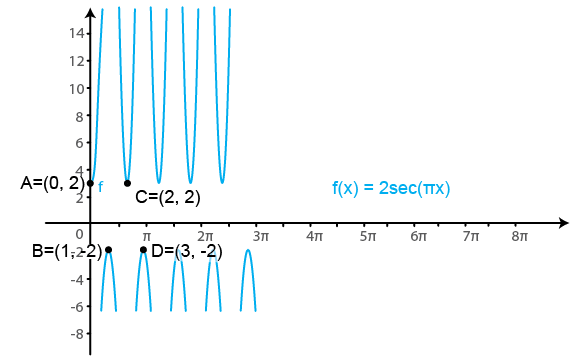Question 5 : f (x) = tan2 x

We know that f (x) = tan x is a periodic function withperiod π.

So, f (x) = tan2 (x) is a periodicfunction with period π. So, we will draw the graph of f (x) = tan2 (x)in the interval [0, π]. The values of f (x) = tan2 (x) atvarious points in [0, π] are listed in the following table:

 x 0 (A) π/2 (B) π/2 (C) π (D) 3π/2 (E) 3π/2 (F) 2 π f (x) = tan2 (x) 0 ∞ →∞ 0 ∞ →∞ 0

The required curve is: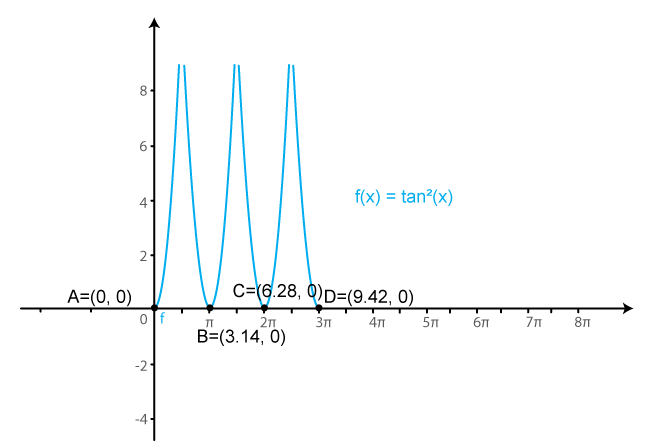Todays Deals### RD Chapter 6- Graphs of Trigonometric Functions Ex-6.3 Contributorskrishan

Name:
Email:

# Latest News# 9000 interview questions in different categories